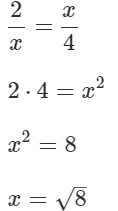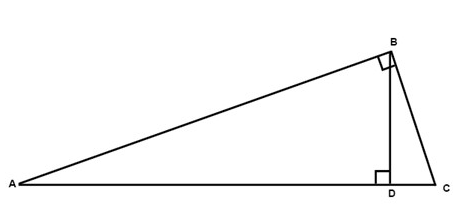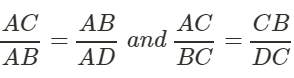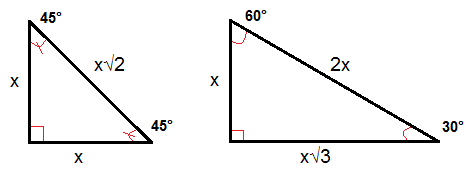Courses

# Right Triangles and Trigonometry Mathematics Notes | EduRev

## Mathematics : Right Triangles and Trigonometry Mathematics Notes | EduRev

The document Right Triangles and Trigonometry Mathematics Notes | EduRev is a part of the Mathematics Course Additional Topics for IIT JAM Mathematics.
All you need of Mathematics at this link: Mathematics

Mean and geometry
The geometric mean is the positive square root of the product of two numbers.
Example
The geometric mean between 2 and 4 is x. The proportion 2:x = x:4 must be true henceIf we in the following triangle draw the altitude from the vertex of the right angle then the two triangles that are formed are similar to the triangle we had from the beginning. The two triangles formed are also similar to each other.△ABC ∼ △BCD ∼ △ABD
The measure of the altitude drawn from the vertex of the right angle to the hypotenuse is the geometric mean between the measures of the two segments of the hypotenuse. Hence BD is the geometric mean of AD and DC.
Also in our figure the measure of a leg of the triangle is the geometric mean between the measures of the hypotenuse and the segment of the hypotenuse adjacent to that leg.The converse of the Pythagorean theorem and special triangles
If we know the sides of a triangle - we can always use the Pythagorean Theorem backwards in order to determine if we have a right triangle, this is called the converse of the Pythagorean Theorem.
If a2 + b2 = c2 then △ABC is a right triangle.
When working with the Pythagorean theorem we will sometimes encounter whole specific numbers that always satisfy our equation - these are called a Pythagorean triple. One common Pythagorean triple is the 3-4-5 triangle where the sides are 3, 4 and 5 units long.
There are some special right triangles that are good to know, the 45°-45°-90° triangle has always a hypotenuse √2 times the length of a leg. In a 30°-60°-90° triangle the length of the hypotenuse is always twice the length of the shorter leg and the length of the longer leg is always √3 times the length of the shorter leg.Offer running on EduRev: Apply code STAYHOME200 to get INR 200 off on our premium plan EduRev Infinity!

40 docs

,

,

,

,

,

,

,

,

,

,

,

,

,

,

,

,

,

,

,

,

,

;